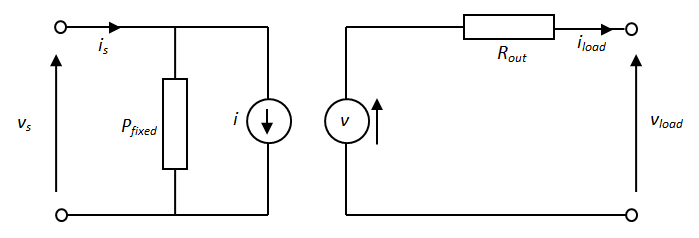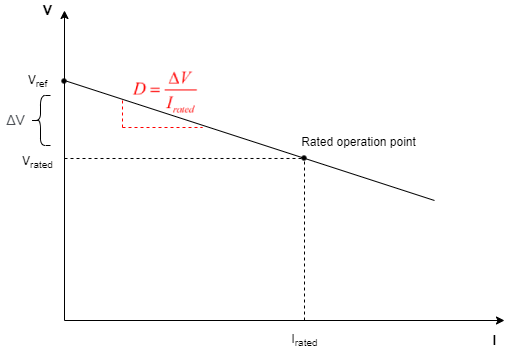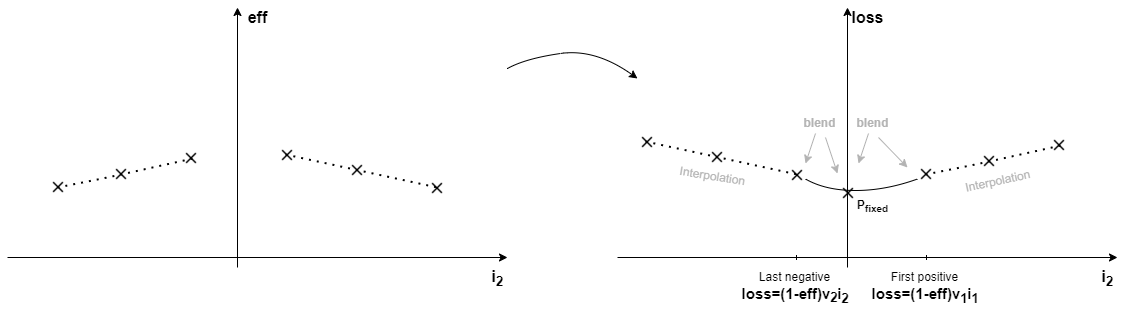# DC-DC Converter

Behavioral model of power converter

•Libraries:
Simscape / Electrical / Semiconductors & Converters / Converters

## Description

The DC-DC Converter block represents a behavioral model of a power converter. This power converter regulates voltage on the load side. To balance input power, output power, and losses, the required amount of power is drawn from the supply side. Alternatively, the converter can support regenerative power flow from load to supply.

This circuit illustrates the converter's behavior.The Pfixed component draws a constant power and corresponds to converter losses that are independent of load current. The power drawn is set by the Converter losses at zero output power parameter value. The resistor Rout corresponds to losses that increase with load current, and is determined from the value you specify for the Percentage efficiency at rated output power parameter.

The voltage source is defined by the following equation:

Where:

• vref is the load side voltage set point, as defined by the value you specify for the Output voltage reference demand parameter. Alternatively, you can provide this value as input to the Vref port when the Voltage reference parameter is set to `External`.

• D is the value you specify for the Output voltage droop with output current parameter. For more information, see Voltage Droop.

The current source value i is calculated so that the power flowing in to the converter equals the sum of the power flowing out plus the converter losses.

To specify the converter behavior when the voltage presented by the load is higher than the converter output voltage reference demand, use the Power direction parameter:

• ```Unidirectional power flow from supply to regulated side``` — Current is blocked by the off-state diode, and the current source current i is zero. Set the conductance of this diode using the Diode off-state conductance parameter.

• `Bidirectional power flow` — Power is transmitted to the supply side, and i becomes negative.

Optionally, the block can include voltage regulation dynamics. If you select `Specify voltage regulation time constant` for the Dynamics parameter, then a first-order lag is added to the equation defining the voltage source value. With the dynamics enabled, a load step change results in a transient change in output voltage, the time constant being defined by the Voltage regulation time constant parameter.

### Voltage Droop

Voltage droop is the loss in output voltage for a device that drives a load. Droop is important in voltage regulation circuits as it increases the tolerance of the systems towards load transients.

A separate value for the droop allows you to control how the output voltage varies with the load regardless of load-dependent losses. To enable droop, select the Enable droop parameter. To specify the droop, set the Droop parameterization parameter to:

• `By voltage droop with output current` — Set the voltage droop directly by specifying the value of the Output voltage droop with output current parameter.

• `By percent voltage droop at rated load` — This equation calculates the voltage droop as a percent of the Output voltage reference demand parameter, based on the value of the Voltage droop percent at rated load parameter:

`$\begin{array}{l}\Delta V=\frac{{D}_{percent}}{100}{V}_{ref_droop}\\ D=\frac{\Delta V\left({V}_{ref_droop}-\Delta V\right)}{{P}_{rated}}\end{array}$`

where `Vref_droop` is the value of the Output voltage reference demand parameter. If you set the Voltage reference parameter to `External`, the block uses the constant value of the Voltage reference used to calculate droop percent parameter to calculate the droop instead.

This plot shows how the block calculates the voltage droop:The voltage droop, D, is the slope of the line, where ${I}_{rated}=\frac{{P}_{rated}}{{V}_{rated}}$ and ${V}_{rated}={V}_{ref_droop}-\Delta V.$

### Tabulated efficiency

You can tabulate the DC-DC Converter block efficiency depending on the output current, input voltage, and temperature.

This equation defines the relationship between losses and efficiency:

`$\begin{array}{l}\begin{array}{cc}loss\left({i}_{2},{v}_{1},T\right)=\left[1-\frac{eff\left({i}_{2},{v}_{1},T\right)}{100}\right]abs\left({v}_{1}{i}_{1}\right)& if\text{\hspace{0.17em}}{i}_{2}<0\text{\hspace{0.17em}}\left(power\text{\hspace{0.17em}}flow\text{\hspace{0.17em}}1\to \text{2}\right)\end{array}\\ \begin{array}{cc}loss\left({i}_{2},{v}_{1},T\right)=\left[1-\frac{eff\left({i}_{2},{v}_{1},T\right)}{100}\right]abs\left({v}_{2}{i}_{2}\right)& if\text{\hspace{0.17em}}{i}_{2}>0\text{\hspace{0.17em}}\left(power\text{\hspace{0.17em}}flow\text{\hspace{0.17em}}2\to \text{1}\right)\end{array}\end{array}$`

where

• loss(i2,v1,T) are the DC-DC converter losses.

• v1 is the input voltage.

• i1 is the input current.

• i2 is the output current.

• T is the device temperature, if you expose the thermal port of this block.

• eff(i2,v1,T) is the efficiency of the DC-DC converter as a function of the output current, input voltage, and temperature, as specified in Percentage efficiency table, eff(I2,V1,T).

If the output current is equal to `0`, the converter losses are equal to Converter losses at zero output power.

These are the steps to compute the used power losses depending on the output current:

1. If the output current is less than the last negative current point or greater than the first positive current point in the Vector of output currents for tabulated efficiencies, I2 parameter, the block uses the Percentage efficiency table, eff(I2) parameter to find the corresponding efficiency (with linear interpolation or nearest extrapolation) and then convert that efficiency to losses.

2. Else, the block blends the zero output current case to the last negative point or the first positive point in the table, as shown in this figure:If you set the Modeling option parameter to ```Show thermal port```, the block tabulates the efficiency depending on the temperature, in addition to the output current and input voltage. The losses calculation remains the same as the option with hidden thermal ports.

### Simulating When the Supply Cannot Match the Load

The DC-DC Converter block uses the Minimum supply voltage to function parameter to protect the network when the voltage supply cannot deliver the load requirements. This happens if you connect a switch in series with the supply and then turn it off, or if the supply connection has a series resistance which drops too much voltage. During the simulation, if the voltage falls below the value of the Minimum supply voltage to function parameter, the block relaxes the regulation of the output voltage. Once the voltage falls below `90`% of the Minimum supply voltage to function parameter, the input of the converter behaves like a fixed resistance equal to the value of the Input resistance if not functioning parameter. Similarly, the output of the DC-DC Converter block behaves like a fixed resistance equal to the value of the Output resistance if not functioning parameter.

### Simulating Faults

You can use the physical signal input port F to simulate a converter failure.

To expose the fault port, F, in the Faults tab, set the Enable output open-circuit fault parameter to `Yes`.

You control the behavior in response to the physical signal fault input F by the parameters on the Faults tab of the block dialog box. With the default parameter settings:

• Fault condition is ```Output open circuit if F >= Fault threshold```

• Fault threshold is `0.5`

If a signal is connected to port F, then the block operates according to the parameter settings on the Faults tab. For example, if Fault condition is ```Output open circuit if F >= Fault threshold```, then when the signal at port F rises above the Fault threshold value, the converter stops operating. Zero current is taken from the supply side, and zero current is supplied to the load side.

### Model thermal effects

This block has one optional thermal port. To expose the thermal port, set the Modeling option parameter to either:

• `No thermal port` — The block does not contain a thermal port.

• `Show thermal port` — The block contains one thermal conserving port.

The block transfers heat generated from electrical losses through a Controlled Heat Flow Rate Source to a Thermal Mass block. The electrical properties of the block do not change with temperature. Specify the thermal properties for this block using the parameters Thermal mass and Initial temperature.

## Assumptions

• The two electrical networks connected to the supply-side and regulated-side terminals must each have their own Electrical Reference block.

• The supply-side equation defines a power constraint on the product of the voltage, vs, and the current, is. For simulation, the solver must be able to uniquely determine vs. To ensure that the solution is unique, the block implements two assertions:

• vs > 0 — This assertion ensures that the sign of vs is uniquely defined

• is < imax — This assertion deals with the case when the voltage supply to the block has a series resistance

When there is a series resistance, there are two possible steady-state solutions for is that satisfy the power constraint, the one with the smaller magnitude being the desired one.

## Ports

### Conserving

expand all

Electrical conserving port associated with the positive terminal of the input side.

Electrical conserving port associated with the negative terminal of the input side.

Electrical conserving port associated with the positive terminal of the output side.

Electrical conserving port associated with the negative terminal of the output side.

Physical signal input port that provides the external voltage reference signal.

#### Dependencies

To enable this port, set the Voltage Reference parameter to `External`.

Physical signal input port that provides the external fault trigger signal.

#### Dependencies

To expose this port, in the Faults tab, set Enable output open-circuit fault to `Yes`.

Thermal conserving port that represents the thermal mass. When you expose this port, provide additional parameters to define battery behavior at a second temperature. For more information, see the Thermal parameters.

#### Dependencies

To enable this port, set Modeling option to ```Show thermal port```.

## Parameters

expand all

Whether to enable the thermal port of the block and model thermal parameters.

### Main

Whether to model the voltage reference internally or externally.

Set point for the voltage regulator, and the output voltage value when there is no output current.

#### Dependencies

This port is visible only when the Voltage Reference parameter is set to `Internal`.

Output power for which the percentage efficiency value is given. This parameter is also used to calculate droop, D, if droop is specified as a percentage.

Whether to enable voltage droop.

Select one of the following methods for droop parameterization:

• ```By voltage droop with output current``` — Specify the absolute value of droop, D. This is the default option.

• ```By percent voltage droop at rated load``` — Specify droop, D, as a percentage at rated load.

#### Dependencies

To enable this parameter, select the Enable droop parameter.

Number of volts that the output voltage will drop from the set point for an output current of 1 A.

#### Dependencies

To enable this parameter, select the Enable droop parameter and set Droop parameterization to ```By voltage droop with output current```.

Percentage by which the voltage drops compared to the nominal output voltage when supplying the rated load. If you set the Voltage reference parameter to `Internal`, the block uses the Output voltage reference demand parameter to calculate the droop percent. If you set the Voltage reference parameter to `External`, the block uses the Voltage reference used to calculate droop percent parameter instead.

#### Dependencies

To enable this parameter, select the Enable droop parameter and set Droop parameterization to ```By percent voltage droop at rated load```.

Voltage reference that the Voltage droop percent at rated load parameter uses to calculate the voltage droop during the simulation.

#### Dependencies

To enable this parameter:

• Set Voltage reference to `External`.

• Select the Enable droop parameter.

• Set Droop parameterization to ```By percent voltage droop at rated load```.

Select one of the following methods for the direction of power conversion:

• ```Unidirectional power flow from supply to regulated side``` — Most small power regulators are unidirectional. This is the default option.

• `Bidirectional power flow` — Larger power converters can be bidirectional, for example, converters used in electric vehicles to allow regenerative braking.

Ideal diode incorporated on the output side to prevent current from being forced into the converter in the unidirectional configuration.

### Losses

Power drawn by the Pfixed component in the equivalent circuit diagram, which corresponds to converter losses that are independent of load current.

Converter losses parameterization.

Efficiency as defined by 100 times the output load power divided by the input supply power.

#### Dependencies

To enable this parameter, set Converter losses to ```Assume proportional to square of output current```.

Whether to tabulate efficiency on the output current, the input voltage, and the temperature. To enable the temperature options, you need to expose the thermal ports of the block.

Vector of output currents for tabulated efficiencies. The size of this parameter must be equal to or greater than `3` and equal to the size of Percentage efficiency table, eff(I).

#### Dependencies

To enable this parameter, set Converter losses to ```Tabulate efficiency```.

Efficiency table in function of the current, in percentage. The size of this parameter must be equal to the size of Vector of output currents for tabulated efficiencies, I2.

#### Dependencies

To enable this parameter, set Converter losses to ```Tabulate efficiency``` and Variables for tabulation to ```Output current```.

Vector of input voltages for tabulated efficiencies. The size of this parameter must be equal to or greater than `2`.

#### Dependencies

To enable this parameter, set Converter losses to ```Tabulate efficiency``` and Variables for tabulation to ```Output current and input voltage``` or ```Output current, input voltage, and temperature```.

Efficiency table in function of the current, in percentage.

#### Dependencies

To enable this parameter, set Converter losses to ```Tabulate efficiency``` and Variables for tabulation to ```Output current```.

Vector of temperatures for tabulated efficiencies. The size of this parameter must be equal to or greater than `2`.

#### Dependencies

To enable this parameter, expose the thermal port of this block and set Converter losses to `Tabulate efficiency`.

Efficiency table in function of current and temperature, in percentage.

#### Dependencies

To enable this parameter, expose the thermal port of this block and set Converter losses to `Tabulate efficiency` and Variables for tabulation to ```Output current and temperature```.

Efficiency table in function of current, voltage, and temperature, in percentage.

#### Dependencies

To enable this parameter, expose the thermal port of this block and set Converter losses to `Tabulate efficiency` and Variables for tabulation to ```Output current, input voltage, and temperature```.

### Dynamics

Specify whether to include voltage regulation dynamics:

• `No dynamics` — Do not consider the voltage regulation dynamics.

• ```Specify voltage regulation time constant``` — Add a first-order lag to the equation defining the voltage source value. With the dynamics enabled, a load step change results in a transient change in output voltage.

Time constant associated with voltage transients when the load current is stepped.

#### Dependencies

To enable this parameter, set Dynamics to ```Specify voltage regulation time constant```.

Value of vref at time zero. Normally, vref is defined by the Output voltage reference demand parameter. However, if you want to initialize the model with no transients when delivering a steady-state load current, you can set the initial vref value by using this parameter, and increase it accordingly to take account of output resistance and droop.

#### Dependencies

To enable this parameter, set Dynamics to ```Specify voltage regulation time constant```.

### Off State

Minimum supply voltage for the converter to operate and regulate the output voltage to the set point. When the supply voltage drops below `90`% of the value of this parameter, the input to the DC-DC Converter block behaves like a fixed resistance equal to the value of the Input resistance if not functioning parameter. Similarly, the output of the DC-DC Converter block behaves like a fixed resistance equal to the value of the Output resistance if not functioning parameter.

Input resistance when the supply voltage falls below `90`% of the value of the Minimum supply voltage to function parameter.

Output resistance when the supply voltage falls below `90`% of the value of the Minimum supply voltage to function parameter.

### Faults

Whether to model output open-circuit faults in the block.

Selects whether the converter is disabled by a signal that is high or low:

• ```Output open circuit if F >= Fault threshold``` — Converter is disabled if the signal at port F rises above the threshold value. This is the default option.

• ```Output open circuit if F <= Fault threshold``` — Converter is disabled if the signal at port F falls below the threshold value.

#### Dependencies

To enable this parameter, set Enable output open-circuit fault to `Yes`.

Threshold value used to detect a fault.

#### Dependencies

To enable this parameter, set Enable output open-circuit fault to `Yes`.

### Thermal

To enable these parameters, set Modeling option to `Show thermal port`.

Thermal mass associated with thermal port H. It represents the energy required to raise the temperature of the thermal port by one degree.

Initial temperature associated with thermal port H.

## Version History

Introduced in R2012b

expand all